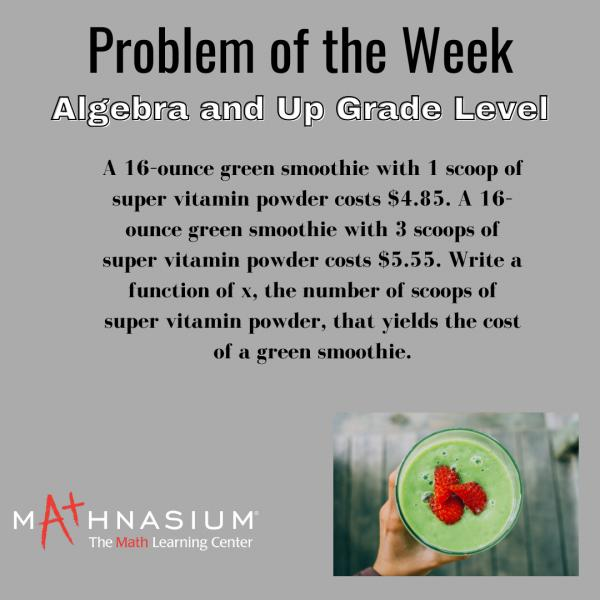* indicates a required field• phone number format invalid• email format invalidProblems detected, please review the form.
Privacy - Terms

# News from Mathnasium of Fair Oaks

### Problem of the Week

Jan 9, 2022# Did you figure it out?

Answer:   f(x) = \$0.35x + \$4.50

Solution:  First, we notice that when the number of scoops of super vitamin powder increases by 2, the price increases by \$5.55 – \$4.85 = \$0.70. So, each scoop of super vitamin powder costs \$0.35. To find the cost of a 16–ounce smoothie without the super vitamin powder, we can subtract \$4.85 – \$0.35 = \$4.50. So, the cost of a green smoothie is \$4.50 plus the total of \$0.35 times the number of scoops:

f(x) = \$0.35x + \$4.50